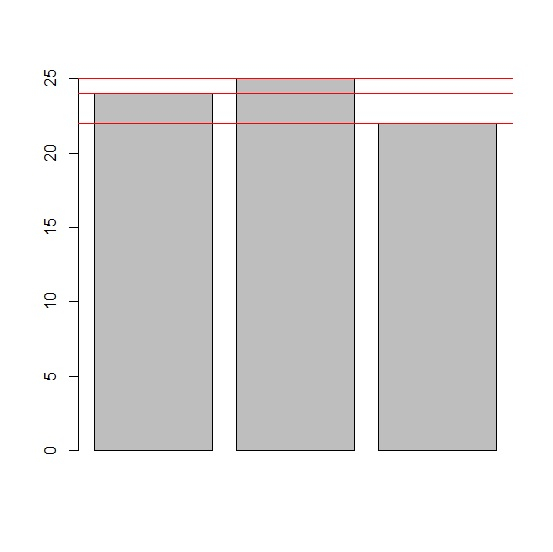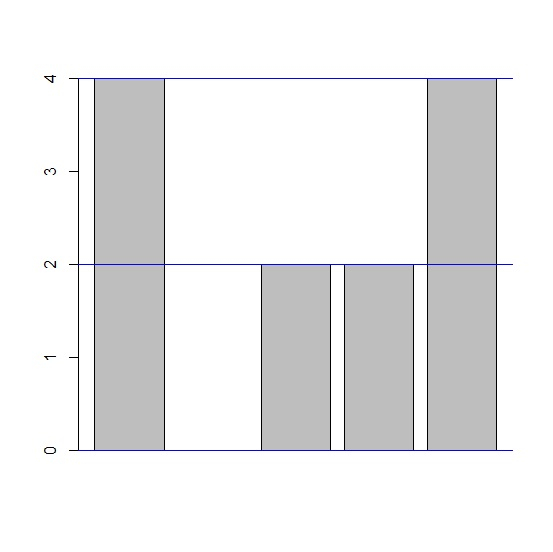# How to create horizontal lines for each bar in a bar plot of base R?

To create horizontal lines for each bar in a bar plot of base R, we can use abline function and pass the same values as in the original barplot with h argument that represents horizontal with different color to make the plot a little better in terms of visualization.

## Example

Live Demo

x<-c(24,25,22)
barplot(x)
abline(h=c(24,25,22),col="red")

## Output## Example2

Live Demo

y<-rpois(5,2)
y

## Output

 4 0 2 2 4

## Example

barplot(y)
abline(h=c(4,0,2,2,4),col="blue")

## Output